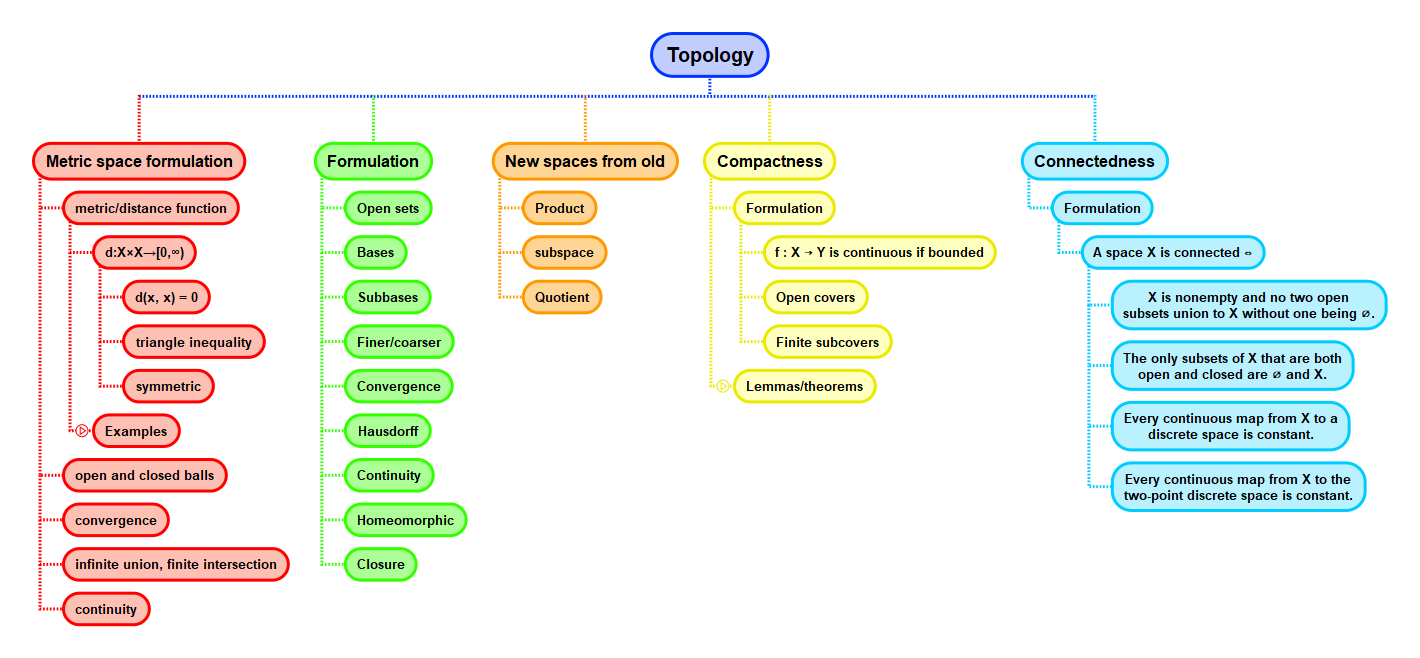Show Question
Math and science::Topology

# Compactness. Subspaces

How do compactness and subspaces interact?

1. A subspace of a compact space is not necessarily compact.
2. Every closed subspace of a compact space is compact.
3. Every compact subspace of a compact space is not necessarily closed.
4. Every compact subspace of a Hausdorff space is closed.
5. A subspace of a compact Hausdorff space is compact iff it is closed.

#### 1. A subspace of a compact space

A subspace of a compact space need not be compact. For example, $$[0, 1]$$ is compact, yet $$(0, 1) \subseteq [0, 1]$$ is not compact. To see this, consider the open cover of $$(0, 1)$$ given by $$((\varepsilon, 1)_{\varepsilon > 0})$$. This cover has no finite subcover. This example highlights that all open covers must have a subcover, and thus, to prove a space to be compact, it is not sufficient to find a single open cover having a finite subcover—we must show that it is impossible for any open cover to not have a finite subcover.

#### 2. Every closed subspace of a compact space is compact.

Proof outline. Any cover of a closed subset $$V$$ can be appended by the single open set $$X \setminus V$$. This new set of subsets must cover $$X$$, and must be finite as $$X$$ is compact.

Proof. Let $$X$$ be a compact space and $$V$$ a closed subset. Let $$(U_i)_{i \in I}$$ be a cover of $$V$$ by open subsets of $$X$$. Then $$(U_i)_{i \in I}$$ together with $$X \setminus V$$ is an open cover of $$X$$. Since $$X$$ is compact, it has some finite subcover; thus, there is some finite $$J \subseteq I$$ such that

$\left( \bigcup_{j \in J} U_j \right) \cup (X \setminus V) = X$

Then $$\bigcup_{j \in J} U_j \subset V$$, as required.

We create an open cover of $$V$$ by subsets of $$X$$, not subsets of $$V$$. This is for convenience, and is justified by the modified formulation of cover for subsets. See Leinster's notes and Lemma B1.7.

The converse is not necessarily true. Which is the topic of the next section.

An idea that appeals to intuition regarding closed vs. open subsets and being compact: closed sets place more stringent requirements on any collection in order for the collection to be a cover. Consequently, some covers that might not have a finite subcover don't count as covers. This conceptualization might help motivate why closed subsets of a compact space are compact, and open subsets are not necessarily.

#### 3. Not all compact subspaces of a compact space is closed.

Trivial counter-examples make this statement false. For example, consider an indiscrete space on a set $$X$$ that has two or more elements. All indiscrete spaces are compact. Now choose a proper subset $$Y \subset X$$ and consider the subspace topology on $$Y$$: the subspace topology on $$Y$$ is indiscrete also (just $$\{Y, \emptyset \}$$), so $$Y$$ is compact. But $$Y$$ is not closed in $$X$$, as it is neither $$\emptyset$$ nor $$X$$.

What other examples are there of non-closed compact subspaces of a compact space are there? Another example is the finite complement topology on $$\mathbb{R}$$. Every subspace of the finite complement topology on $$\mathbb{R}$$ is compact (try justify!). But the only proper subsets of $$\mathbb{R}$$ that are closed in this topology are the finite sets.

With a pretty minimal extra restriction on $$X$$ (being Hausdorff), we can make the desired assertion.

#### 4. Every compact subspace of a Hausdorff space is closed.

Note first that we have relaxed the requirement that the larger/outer space be compact.

Proof. Let $$Y$$ be a compact subspace of the Hausdorff space $$X$$. We shall prove that $$X - Y$$ is open, so that $$Y$$ must be closed. Let $$x_0 \in X - Y$$.

Claim: there is a neighbourhood of $$x_0$$ that is disjoint from the whole of $$Y$$.

Claim proof. Since $$X$$ is Hausdorff, for each $$y \in Y$$, with respect to $$x_0$$ choose disjoint neighbourhoods $$U_y$$ and $$U_{x_0}$$: in other words $$U_y$$ is a neighbourhood of $$y$$ and $$U_{x_0}$$ is a neighbourhood of $$x_0$$. The collection $$\{U_y : y \in Y \}$$ is a covering of $$Y$$ by sets open in $$X$$. [This next bit is the one and only point where we utilize the compactness of $$Y$$.] There must be a finite sub-cover, $$U_Y = U_{y_1}, U_{y_2}, ..., U_{y_n}$$. This finite cover $$U_Y$$ contains $$Y$$ and is disjoint from $$x_0$$ and its neighbourhood $$Ux_0 = U_1, U_1, U_n$$, formed by taking the finite intersection of neihbourhoods disjoint from each of the neigbourhoods that were unioned to form $$U_Y$$. This proofs our claim.

Now we have a neighbourhood of $$x_0$$ disjoin from $$Y$$. This is true for any $$x \notin Y$$, so we may take the union of such open sets which will in turn be open. Let $$W$$ be this open set. We have $$X - W = Y$$, so $$Y$$ must be closed. This completes the whole proof.

The finite complement topology was used as an example for #3. The finite complement topology is not a Hausdorff space, as any two neighbourhoods always overlap; we then fail trying to setup a neighbourhood around $$x_0$$ disjoint from $$Y$$. This highlights the difference between #3 and #4).

#### Lemma: 'neighbourhood' of a compact subspace

If $$Y$$ is a compact subspace of a Hausdorff space $$X$$, and $$x_0$$ is not in $$Y$$, then there exists disjoin open sets $$U_{x_0}$$ and $$U_Y$$ of $$X$$ such that $$U_{x_0}$$ contains $$x_0$$ and $$U_Y$$ contains $$Y$$.

This lemma is the inner claim from the proof above. Munkres makes the point this this lemma is a useful result in its own right. He even provides a nice diagram (see figure 26.1 in the text).

#### A subspace of a compact Hausdorff space is compact iff it is closed.

This is the combination of 2 & 4.

## ContextMunkres p164